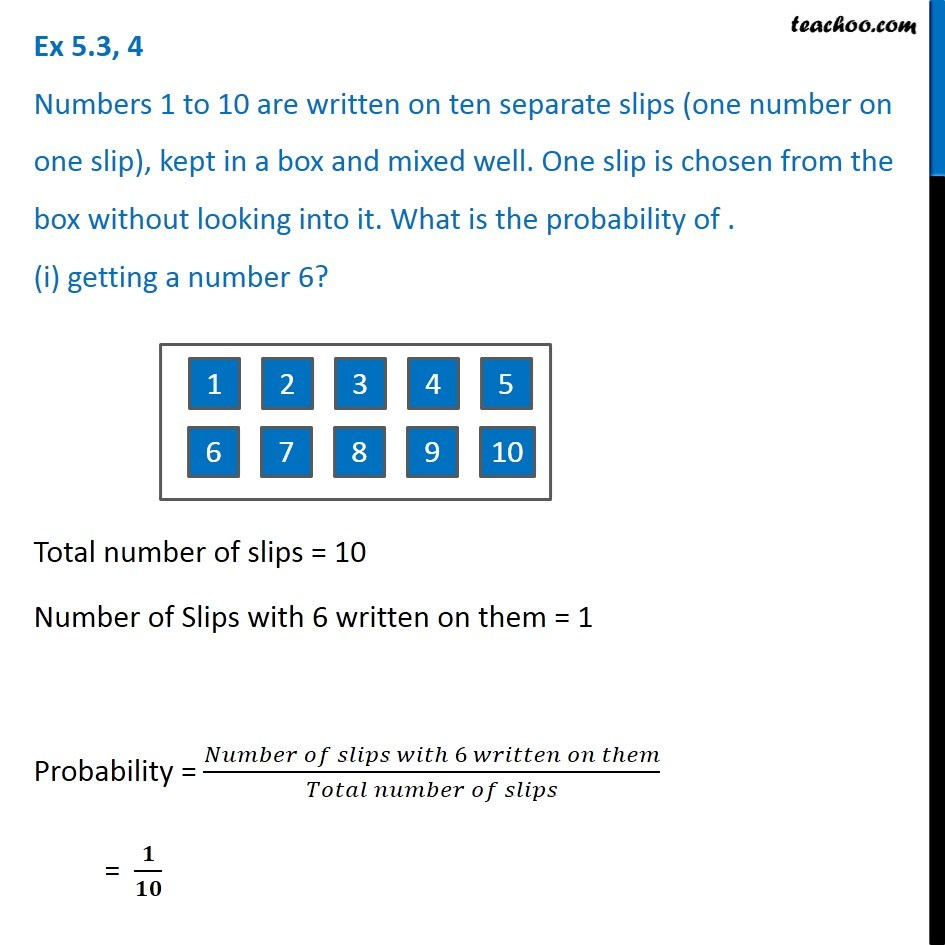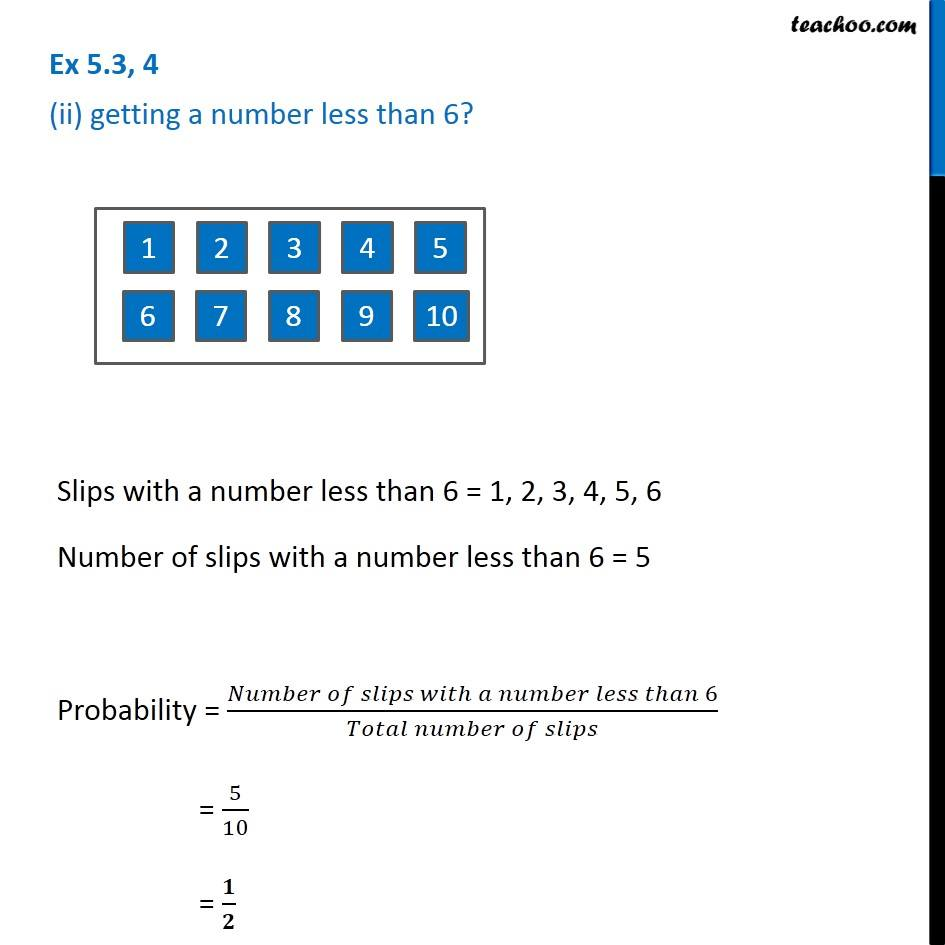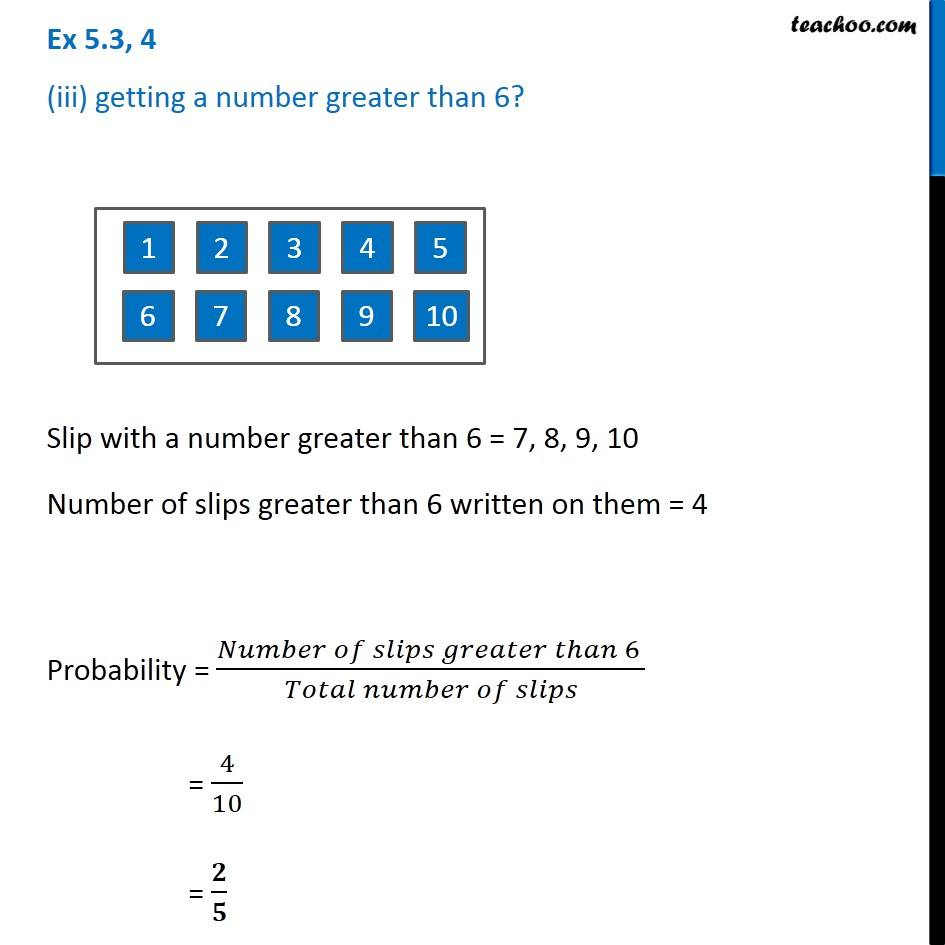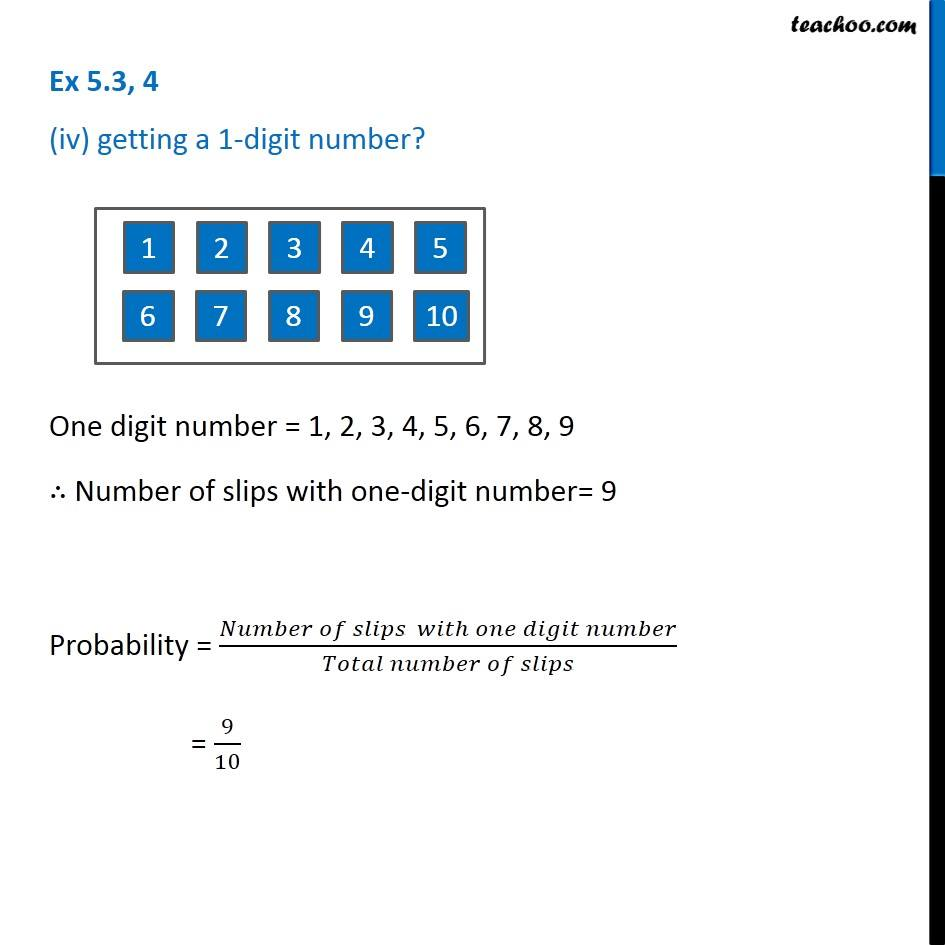Probability

Chapter 5 Class 8 Data Handling
Concept wiseIntroducing your new favourite teacher - Teachoo Black, at only ₹83 per month

### Transcript

Ex 5.3, 4 Numbers 1 to 10 are written on ten separate slips (one number on one slip), kept in a box and mixed well. One slip is chosen from the box without looking into it. What is the probability of . (i) getting a number 6? Total number of slips = 10 Number of Slips with 6 written on them = 1 Probability = (𝑁𝑢𝑚𝑏𝑒𝑟 𝑜𝑓 𝑠𝑙𝑖𝑝𝑠 𝑤𝑖𝑡ℎ 6 𝑤𝑟𝑖𝑡𝑡𝑒𝑛 𝑜𝑛 𝑡ℎ𝑒𝑚)/(𝑇𝑜𝑡𝑎𝑙 𝑛𝑢𝑚𝑏𝑒𝑟 𝑜𝑓 𝑠𝑙𝑖𝑝𝑠) = 𝟏/𝟏𝟎 Ex 5.3, 4 (ii) getting a number less than 6? Slips with a number less than 6 = 1, 2, 3, 4, 5, 6 Number of slips with a number less than 6 = 5 Probability = (𝑁𝑢𝑚𝑏𝑒𝑟 𝑜𝑓 𝑠𝑙𝑖𝑝𝑠 𝑤𝑖𝑡ℎ 𝑎 𝑛𝑢𝑚𝑏𝑒𝑟 𝑙𝑒𝑠𝑠 𝑡ℎ𝑎𝑛 6)/(𝑇𝑜𝑡𝑎𝑙 𝑛𝑢𝑚𝑏𝑒𝑟 𝑜𝑓 𝑠𝑙𝑖𝑝𝑠) = 5/10 = 𝟏/𝟐 Ex 5.3, 4 (iii) getting a number greater than 6? Slip with a number greater than 6 = 7, 8, 9, 10 Number of slips greater than 6 written on them = 4 Probability = (𝑁𝑢𝑚𝑏𝑒𝑟 𝑜𝑓 𝑠𝑙𝑖𝑝𝑠 𝑔𝑟𝑒𝑎𝑡𝑒𝑟 𝑡ℎ𝑎𝑛 6 )/(𝑇𝑜𝑡𝑎𝑙 𝑛𝑢𝑚𝑏𝑒𝑟 𝑜𝑓 𝑠𝑙𝑖𝑝𝑠) = 4/10 = 𝟐/𝟓 Ex 5.3, 4 (iv) getting a 1-digit number? One digit number = 1, 2, 3, 4, 5, 6, 7, 8, 9 ∴ Number of slips with one-digit number= 9 Probability = (𝑁𝑢𝑚𝑏𝑒𝑟 𝑜𝑓 𝑠𝑙𝑖𝑝𝑠 𝑤𝑖𝑡ℎ 𝑜𝑛𝑒 𝑑𝑖𝑔𝑖𝑡 𝑛𝑢𝑚𝑏𝑒𝑟)/(𝑇𝑜𝑡𝑎𝑙 𝑛𝑢𝑚𝑏𝑒𝑟 𝑜𝑓 𝑠𝑙𝑖𝑝𝑠) = 9/10